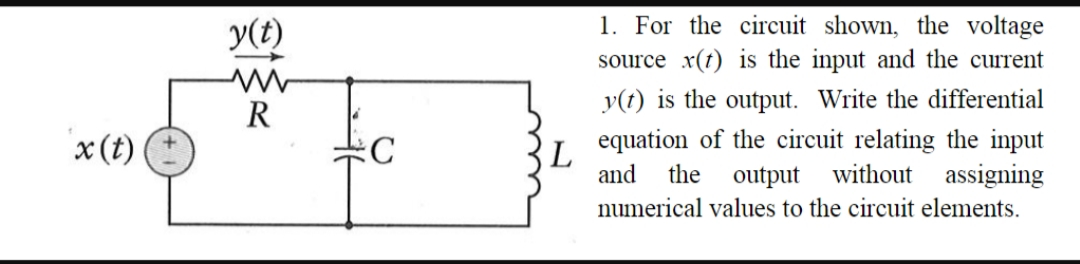# 1. For the circuit shown, the voltageУ()source x(t) is the input and the currenty(t) is the output. Write the differentialRequation of the circuit relating the inputthe output without assigningnumerical values to the circuit elementsx(t)СLand

Question
85 viewshelp_outlineImage Transcriptionclose1. For the circuit shown, the voltage У() source x(t) is the input and the current y(t) is the output. Write the differential R equation of the circuit relating the input the output without assigning numerical values to the circuit elements x(t) С L and fullscreen
check_circle

Step 1

Draw a schematic circuit diagram,

Step 2

Calcalate the voltage across the resistor,

Step 3

Calculate the voltage acr...

### Want to see the full answer?

See Solution

#### Want to see this answer and more?

Solutions are written by subject experts who are available 24/7. Questions are typically answered within 1 hour.*

See Solution
*Response times may vary by subject and question.
Tagged in

### Electrical Engineering